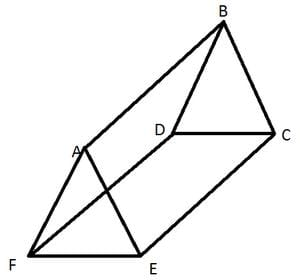Test: Visualising Solid Shapes

# Test: Visualising Solid Shapes

Test Description

## 20 Questions MCQ Test Mathematics (Maths) Class 7 | Test: Visualising Solid Shapes

Test: Visualising Solid Shapes for Class 7 2022 is part of Mathematics (Maths) Class 7 preparation. The Test: Visualising Solid Shapes questions and answers have been prepared according to the Class 7 exam syllabus.The Test: Visualising Solid Shapes MCQs are made for Class 7 2022 Exam. Find important definitions, questions, notes, meanings, examples, exercises, MCQs and online tests for Test: Visualising Solid Shapes below.
Solutions of Test: Visualising Solid Shapes questions in English are available as part of our Mathematics (Maths) Class 7 for Class 7 & Test: Visualising Solid Shapes solutions in Hindi for Mathematics (Maths) Class 7 course. Download more important topics, notes, lectures and mock test series for Class 7 Exam by signing up for free. Attempt Test: Visualising Solid Shapes | 20 questions in 20 minutes | Mock test for Class 7 preparation | Free important questions MCQ to study Mathematics (Maths) Class 7 for Class 7 Exam | Download free PDF with solutions
 1 Crore+ students have signed up on EduRev. Have you?
Test: Visualising Solid Shapes - Question 1

### The number of faces of a sphere is _______.

Detailed Solution for Test: Visualising Solid Shapes - Question 1

Sphere has only one face which is the only circular surface

Test: Visualising Solid Shapes - Question 2

### The number of vertices of a cube is _______.

Test: Visualising Solid Shapes - Question 3

### The number of vertices of a cone is _______.

Test: Visualising Solid Shapes - Question 4

The number of faces of a triangular prism is _______.

Detailed Solution for Test: Visualising Solid Shapes - Question 4

A triangular prism has 2 triangles and 3 rectangles. The one with 4 faces is triangular pyramid.

Test: Visualising Solid Shapes - Question 5

The number of faces of a square pyramid is _______.

Test: Visualising Solid Shapes - Question 6

The number of faces of a triangular pyramid or tetrahedron is _______.

Test: Visualising Solid Shapes - Question 7

The number of triangular faces of a triangular prism is _______.

Detailed Solution for Test: Visualising Solid Shapes - Question 7Test: Visualising Solid Shapes - Question 8

The number of rectangular faces of a triangular prism is _______.

Detailed Solution for Test: Visualising Solid Shapes - Question 8

A triangular prism has 3 rectangular faces joining the two triangles.

Test: Visualising Solid Shapes - Question 9

The number of triangular faces of a rectangular pyramid is _______.

Test: Visualising Solid Shapes - Question 10

Which of the following is the number of vertices of sphere?

Test: Visualising Solid Shapes - Question 11

The number of edges of a triangular prism is _______.

Test: Visualising Solid Shapes - Question 12

The number of edges of a square pyramid is _______.

Detailed Solution for Test: Visualising Solid Shapes - Question 12

The square base has 4 edges and all the vertices joining one vertex at the top form 4 more edges which totals to 8.

Test: Visualising Solid Shapes - Question 13

The number of edges of a triangular pyramid is _______.

Test: Visualising Solid Shapes - Question 14

The number of edges of a rectangular pyramid is _______.

Test: Visualising Solid Shapes - Question 15

The number of faces of a triangular prism is _______.

Detailed Solution for Test: Visualising Solid Shapes - Question 15

A triangular prism consists of 2 triangles and 3 rectangles. So it has two triangular faces and three rectangular faces. So it has 5 faces.

Test: Visualising Solid Shapes - Question 16

The number of faces of a triangular pyramid is _______.

Test: Visualising Solid Shapes - Question 17

The number of faces of a square pyramid is _______.

Test: Visualising Solid Shapes - Question 18

The number of faces of a rectangular prism is _______.

Test: Visualising Solid Shapes - Question 19

The corners of a solid shape are called its ________.

Detailed Solution for Test: Visualising Solid Shapes - Question 19

The corners of a solid shape are called its vertices; the line segments of itsskeleton are its edges; and its flat surfaces are its faces.

Test: Visualising Solid Shapes - Question 20

A _______ is a skeleton-outline of a solid that can be folded to make it

## Mathematics (Maths) Class 7

168 videos|252 docs|45 tests
 Use Code STAYHOME200 and get INR 200 additional OFF Use Coupon Code
Information about Test: Visualising Solid Shapes Page
In this test you can find the Exam questions for Test: Visualising Solid Shapes solved & explained in the simplest way possible. Besides giving Questions and answers for Test: Visualising Solid Shapes, EduRev gives you an ample number of Online tests for practice

## Mathematics (Maths) Class 7

168 videos|252 docs|45 tests